Jim Worthey • Lighting & Color Research • jim@jimworthey.com • 301-977-3551 • 11 Rye Court, Gaithersburg, MD 20878-1901, USA
 Computer Programming Examples for Vectorial Color
 0 Jump directly to program listings. 1 On this web site, the phrase "vectorial color" denotes a set of methods in which lights are represented by vectors in the color space developed by Jozef Cohen. The vectors are tristimulus vectors, based on the orthonormal color matching functions. The goal is to make better use of existing color-matching data. Whether the 10° data are better than the 2° data, or new experimental data are needed, those are separate questions. You choose your data, and the vectorial methods will help to make use of them. The starting point for vectorial color is to take existing color matching functions, such as the 2° observer functions, and add and subtract them to make orthonormal color matching functions. The "adding and subtracting" is done by matrix algebra and in one step uses the well-known Gram-Schmidt method of orthonormalization. The purpose of this web page (as of 2006 June) is to present a few code fragments and short working routines, to show how the work is done. With regard to some methods, such as Gram-Schmidt, it is easier to write a program than to describe the method in words and symbols. I do all my calculations in a science-programming language called O-Matrix, which allows matrix operations to be written as easily as operations on scalars. If a and b are matrices previously created, one can write c = a*b to right-multiply a by b. The matrix c need not be declared or dimensioned in advance. In short, steps that are simple in concept remain simple in the computer program. Another language similar to O-Matrix is Matlab. The O-Matrix manual exists only as web pages, and you can view a version on their web site. If you would like to discuss color calculations, or request more details, please contact jim@jimworthey.com , or call 301-977-3551 in the USA.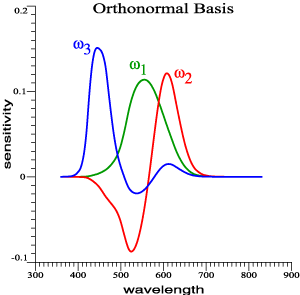2 Suppose for a moment that the orthonormal color matching functions have been found. For the 2° observer, they are as graphed above. Conceptually, they can be 3 column matrices, ω1, ω2, ω3. The three column vectors can be grouped together in an N×3 matrix, where N is the number of wavelengths: Ω = [ω1 ω2 ω3]  . This is the kind of notation used in the articles, with the Greek letters, big and little omega. For programming, it is convenient to call Ω something like OrthoBasis: Ω → OrthoBasis  . If an individual vector is needed, it can be referred to in O-Matrix as OrthoBasis.col(j), where j = 1, 2, or 3 as needed. The notation ".col(2)" selects the second column of a matrix, for example. Now suppose that D65 is a column vector representing the spectrum of standard daylight, D65. We wish to calculate its tristimulus vector, which can be called V65. Then V65 = OrthoBasis'*D65   . That line of code is in a larger font to be sure that you can see the apostrophe after OrthoBasis. It indicates matrix transpose. Using the proper notation then, the tristimulus vector of any light can be found in one line of computer code. In order to do the operations of colorimetry by matrix operations, one must align the functions of wavelength properly: they must have the same number of elements and the same wavelength domain.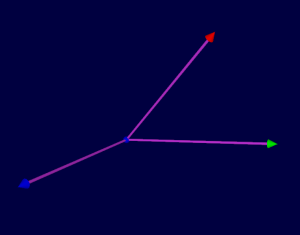3 In the visuals of the 2004 talk, "Color Matching with Amplitude not Left Out," or in the new unpublished article, "Vectorial Color," the idea of orthonormal color matching functions is developed step-by-step. It is logical to have one all-positive function, and to let that be an achromatic function that is proportional to the usual y-bar. But the achromatic function can be normalized. Suppose that ybar is usual 2° observer function. Then a code fragment will show the steps: prod = ybar'*ybar omega1 = ybar/sqrt(prod)  . Again the apostrophe denotes matrix transpose. sqrt(prod) is then the vector length of ybar. Dividing ybar by its length gives omega1, a column matrix proportional to ybar, but with length = 1. To verify, print omega1'*omega1  . The result should equal one. Now suppose that red is a red cone sensitivity function. We know that omega1 is a sum of red and green, with certain proportions. To make an opponent function orthnormal to omega1, we subtract from red the projection of omega1 onto red, then normalize: omega2 = red - (red'*omega1)*omega1 prod = omega2'*omega2 omega2 = omega2/sqrt(prod) In normal programming fashion, the array omega2 holds an intermediate matrix, then the final answer. The steps above illustrate the method of Gram-Schmidt orthonormalization. The following O-Matrix routine calculates an orthonormal set from the columns of any matrix: GramSchmidt(given, orthonorm)  . The link will bring up a text file containing the program. To run it, you must save the text in a file called GramSchmidt.oms. Calling the Gram-Schmidt routine by the following steps will create OrthoBasis: given = [ybar, red, blue] NumCols = 3 NumRows = rowdim(given) OrthoBasis = zeros(NumRows,NumCols) GramSchmidt(given, OrthoBasis) It is assumed that there is a set of cone functions {red, green, blue} that are linear combinations of the usual xbar, ybar, zbar of the 2° observer, and indeed that ybar is a linear combination of red and green only. For background, see the Vectorial Color manuscript, especially the appendices and Figure 1. Also see item 4 below.4 Just above, and in Figure 1 of the Vectorial Color Manuscript, reference is made to cone sensitivity functions that are linear combinations of the usual 2° observer. I take the idea from Guth, who borrowed it from Smith and Pokorny. Guth's formula for the cone functions is recalled in Appendix A of the "Render Calc" article. Taking the matrix transpose of that version, including a transposed version of the square matrix, we can write in O-Matrix: M1 = { [0.2435, -0.3954, 0], ... [0.8524, 1.1642, 0], ... [-0.0516, 0.0837, 0.6225] } rgb = xyzbar*M1 The columns of xyzbar are assumed to be the 2° observer functions. That is xyzbar = [xbar, ybar, zbar] . The actual data are here, or can be obtained from www.cvrl.org . For my work, I store most spectral data in a particular format that is convenient, but here the xyzbar data are lumped in one file, just to show that they're available. To get Guth's 1980 opponent model directly from xyzbar, M3 = {[0, 0.7401, -0.0061], ... [0.9341, -0.6801, -0.0212], ... [0, -0.1567, 0.0314] } atd  = xyzbar*M3 The Guth functions can then be orthonormalized in one step: OrthoBasis = zeros(NumRows,NumCols) GramSchmidt(atd, OrthoBasis) The result is the same orthonormal basis as in item 3. The result should also check against this file.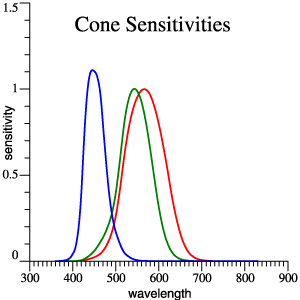5 Matrix R. Let A be a matrix whose columns are three linearly independent vectors, such as three color matching functions. For example, A could be the usual  2° observer functions. Then in O-Matrix, projection matrix R can be found by Cohen's formula: A = xyzbar R = A*inv(A'*A)*A' In the special case that the columns of A are an orthonormal set, for example if A = OrthoBasis, then A'*A is an identity matrix. Its inverse is also an identity matrix and one may write for this special case only, R =  OrthoBasis*OrthoBasis' An O-Matrix function can be used: Matrix R is a large matrix. For example, if A indeed contains the 2° observer functions at 1 nm intervals and wavelength domain 360 to 830 nm, then R has dimensions 471×471, meaning that it has 221841 elements and in double precision (8 bytes per floating point number), it occupies 1774728 bytes. On modern PC hardware, this storage space and the time to calculate R are absolutely not a concern. However, it is not helpful to print out the matrix on paper. On my PC, the time to calculate a  471×471 matrix R by function RCohen() is about 13 ms.6 Putting Matrix R to work. The projection matrix R can be viewed in 2 ways. For routine use of the orthonormal basis, matrix R is not needed at all. Matrix R can be considered as background information, as the proof that the locus of unit monochromats has an invariant shape, independent of the coordinate system. The orthonormal basis is a shortcut for creating color vectors without using matrix R itself. On the other hand, matrix R can be a practical tool for curve-fitting problems that arise in relation to color. If matrix A contains any 1, 2, 3, or more linearly independent vectors, then the projection matrix R can be found. Then if any vector X (with the same number of rows as A or R) is multiplied by R, the result is the projection of X into the column space of A. That is, Xstar = R*X, where Xstar is the projection. An alternate wording is that Xstar is the least-squares best fit to X, using the columns of A. On the right are graphed the red, green, and blue sensitivities of a digital camera. In analyzing and applying this color sensor, one might wish to find a linear combination of the camera's red and green sensitivities that best approximates the human achromatic sensitivity. An alternate statement of the same goal is that we wish to project the human achromatic function into the the vector space of the camera's red and green sensors. Let the camera's sensitivities be called redcam, greencam, bluecam. Then Arg = [redcam, greencam] Rrg = RCohen(Arg) achcam = Rrg*OrthoBasis.col(1) Column matrix achcam is then the best approximation to the human achromatic function, OrthoBasis.col(1), by a combination of redcam and greencam. The lower graph shows achcam compared to OrthoBasis.col(1). More steps would be needed for the complete analysis of a camera. At this moment, in 2006 June, I am preparing a paper on that topic, with a co-author. For now, the topic is calculation. The methods on this page can be used for such steps as these: Find an orthonormal basis for the camera itself. By combining those functions, find the camera's locus of unit monochromats. Find the best fit to all 3 human sensitivities by the camera sensitivities. The actual programming is reduced to a few steps, and the problem becomes one of concepts: what do you want the camera to do?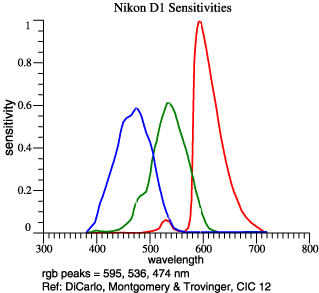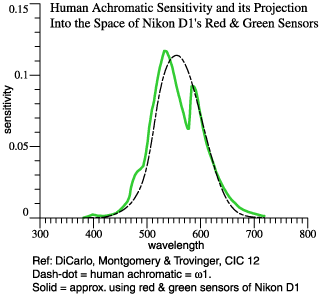7 Blackbody spectra can be calculated by this routine: blackbody(Tkelvin, descrip, LamLoHi, vec)  . Tkelvin = Kelvin temperature of the blackbody descrip = a character array that will be set with a description of this light LamLoHi = the domain of wavelength, such as [360, 830]. vec = the result, a column matrix containing the blackbody spectrum The blackbody spectrum is adjusted so that it = 1 at 555 nm. In this case, LamLoHi  is an input, set by the calling program. My programs are inconsistent about this, whether LamLoHi must be set by the calling routine. Since I want all spectra to have the same domain, the domain is often set by a global variable.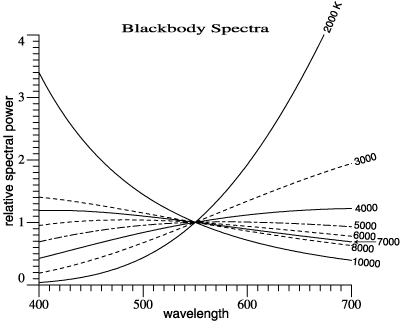8
 8½
 9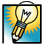web site designed by Nick Worthey